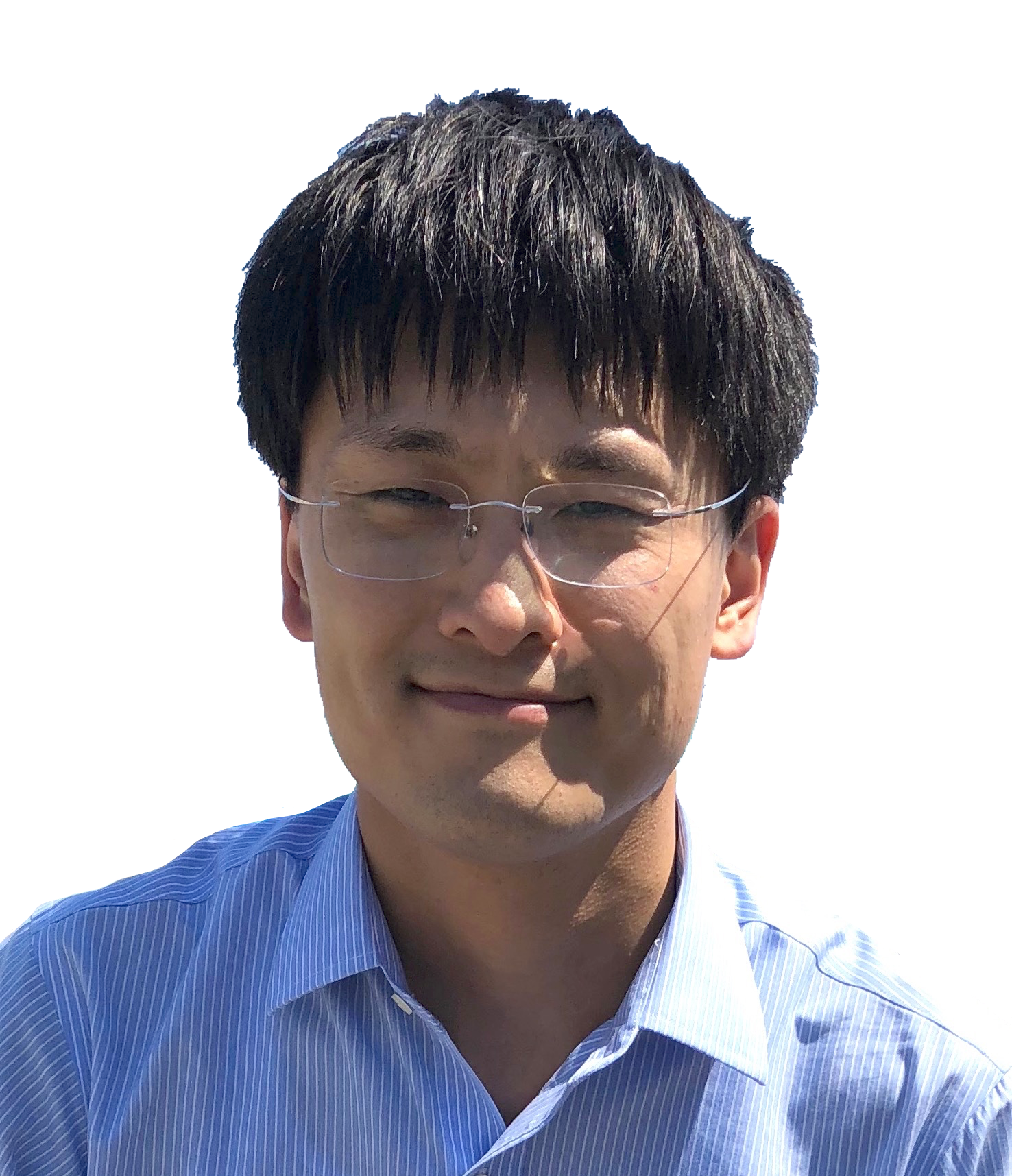DocumentProfessor and RGC Research Fellow
Department of Applied Mathematics
The Hong Kong Polytechnic University
Hung Hom, Hong Kong
https://orcid.org/0000-0001-7566-3464

### Research Interests     Publication     Research Group     PhD and Postdoctoral Positions

• Numerical methods and analysis for partial differential equations, including
• Surface evolution under geometric flows, geometric evolution equations, PDEs on surfaces ← click here
• Low-regularity approximation to nonlinear dispersive equations
1. An unfiltered low-regularity integrator for the KdV equation with solutions below H1 (PDF)
2. A constructive low-regularity integrator for the 1D cubic nonlinear Schrödinger equation under the Neumann boundary condition (PDF)
3. A fully discrete low-regularity integrator for the 1D periodic cubic nonlinear Schrödinger equation (PDF)
4. A second-order low-regularity correction of Lie splitting for the semilinear Klein-Gordon equation (PDF)
• Incompressible Navier–Stokes equations
• Semilinear parabolic equations and phase field equation
• Interior penalty finite element methods and perfectly matched layer (PML) for the Helmholtz equation
• Maximal Lp-regularity of time discretization methods for parabolic equations
• Maximum-norm stability and maximal Lp-regularity of finite element methods
• High-order approximation of singular solutions of fractional evolution equations
• Dynamic Ginzburg–Landau superconductivity equations in nonsmooth domains
• Time-dependent Joule heating problem (for thermistors with temperature-dependent electric conductivity (PDF)
• ### Editorial boards

• Mathematics of Computation:                 2022.2 –– present

• SIAM Journal on Numerical Analysis:   2022.1 –– present

### Awards

• RGC Research Fellow Award 2023 (by the Hong Kong Research Grants Council).

• The Hong Kong Mathematical Society Young Scholars Award 2022.

• Alexander von Humboldt Research Fellowship, 2015-2016.

• Best Paper Award for Young Scholars 2013, The Chinese Society for Computational Mathematics
(于第十二届全国高校计算数学年会).